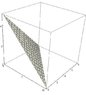# Triple Integral

• Larrytsai

## Homework Statement

Evaluate the triple integral of xy*dxdydz where E is the solid tetrahedon with vertices (0,0,0) (10,0,0) (0,8,0) (0,0,5).

## The Attempt at a Solution

Im trying to integrate dx and dy with bounds from 0 to the line that describes them with respect to the z axis,

so for

dy i have the bounds as

0 to y= -8z/5 + 8

dx

0 to x= -2z + 10

and dz

0 to 5

will this work?

I don't think so. I'll give you a suggestion but you may not like it: first solve it via dzdydx because that's more natural and helps you understand the process then you can use what you learn to solve it via dxdydz. But first, you'd have to determine the equation of the plane that is the "top" of the tetrahedron (see below) and that involves computing the cross product to obtain the normal to the plane. Once you do that, then the integral is easy and can be represented as:

$$V=\int_0^{10}\int_0^{f(x)}\int_{0}^{g(x,y)} xy dzdydx$$

#### Attachments

•mytet.jpg
27.4 KB · Views: 328